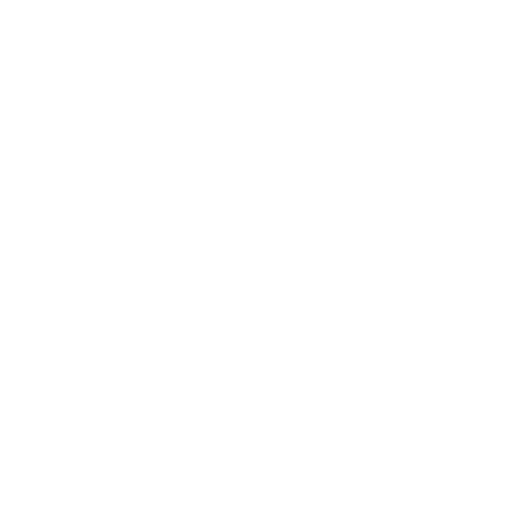# P.# Building Predictable State Containers

I always want to make my blog posts more entertaining. Should I be more funny? Should I be clever? Should I be cool? I'm not cool. I'm just going to keep this post short and to the point.

### What is a predictable state container?

Predictable state containers are made up of two things: an observable and a reducer. The observable is the container itself and the reducer manages state. State changes within the observable are predictable because all state transformations are made by applying the old state and an action to a pure function (i.e the reducer).

Here's an example of a predictable state container, in just a few lines of code.

``````function Observable(reducer, initialState) {
let currentState = initialState
let listeners = []

let subscribe = (listener) => listeners.push(listener)

let dispatch = (action) => {
currentState = reducer(currentState, action)

listeners.forEach(listener => listener(currentState))
}

return { subscribe, dispatch }
}
``````

A seasoned javascript developer might recognise this observable, it's redux! And an even more seasoned developer might recognise a paradigm, it's reactive programming.

There's always more than one solution to a given problem. What if redux didn't exist? How would you build an observable state container?

I'm not going to go into the details of defining reactive programming in this blog post, instead I want to borrow the core principles of Redux (a single source of truth, read-only state and changes made with pure function), and look for different ways to build predictable state containers.

Let's go back to what a state container is made up of (an observable and a reducer) and approach the problem from two extremes, using some popular libraries.

### Building a state container using RxJs

RxJs is everything you could possible want out of observables. It's set of libraries for composing observable sequences (sometimes referred to as "data streams"). The source of the data stream is event based. It could be: a click event, an ajax requests, an update to a data store, etc.

Let's take a look at how we would build a predictable state container using RxJs. In the example below we're building a counter.

``````var Rx = require('rx')

// First we define a "subject", this will act as our
// store's dispatcher. A subject is an object that is
// both an observable sequence as well as an observer.

var subject = new Rx.Subject()

// Next we define our actions, by filtering the data streams
// by actions.type than we map the action to a pure function.

var incReducer = subject
.filter(a => a.type === 'INC')
.map(a => s => s + 1)

var decReducer = subject
.filter(a => a.type === 'DEC')
.map(a => s => s - 1)

// Next, we define our store's initial state.
var initialState = 0;

// Lastly, we compose our actions (i.e observable sequences) into
// a single observable (the data store)

var store = Rx.Observable
.merge(incReducer, decReducer)
.startWith(initialState)
.scan((x, f) => f(x))

store.subscribe((s) => {
console.log(s)
})

// When we call `onNext` the subject will return a pure function
// associated with the action.type, and the store will use that
// pure function to reduce the current state by calling `scan`(a derivative of `reduce`).

subject.onNext({ type: 'INC' }) // => 1
subject.onNext({ type: 'INC' }) // => 2
subject.onNext({ type: 'DEC' }) // => 1
``````

### Building a state container using Ramda

Ramda is everything you could want out of functional programming. Functions in Ramda are automatically curried and it has an emphasises on a purer functional style of programming.

I think it would be interesting to use transducers to build abstract actions for our data store.

A transducer is function that accepts a reducer and returns a reducer (i.e a pure function).

So let's do this!

``````var R = require('ramda')
var reduceReducers = require('reduce-reducers')
var Container = require('./ramda-container') // see github source

// First, we define our actions.
// actions will have the following api:
// f(state, actions) they're reducers!

var increment = transduce(
R.filter(a => a.type === 'INC'),
)

var decrement = transduce(
R.filter(a => a.type === 'DEC'),
R.compose(R.flip(R.subtract)(1))
)

// Next, we combine our actions into a single reducer

var rootReducer = reduceReducers(increment, decrement)

var subject = (new Container)
.initialState(0)
.reducer(rootReducer)
.subscribe((s) => {
console.log(s)
})

// When we call `onNext` the action is applied to the root reducer
// to update the store's state.

subject.onNext({ type: 'INC' }) // => 1
subject.onNext({ type: 'INC' }) // => 2
subject.onNext({ type: 'DEC' }) // => 1
``````

#### Source Code

https://github.com/paulserraino/state-containers